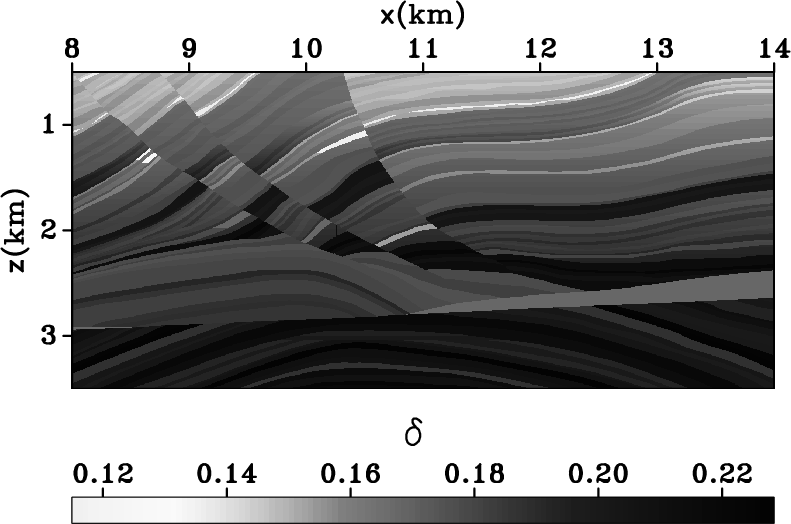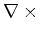Elastic wave-mode separation for TTI mediaNext: 3D TTI model Up: Examples Previous: 2D TTI fold model

## Marmousi II model

My second model (Figure 12) uses an elastic anisotropic version of the Marmousi II model (Bourgeois et al., 1991). In the modified model,is taken from the original model (Figure 12(a)), theratio ranges from 2 to 2.5, (Figure 12(b)), and the densityis taken from the original model (Figure 12(c)). The parameterandare derived from the density modelwith the relations ofand, respectively. The parameterranges fromtoFigure 12(d), and parameterranges fromtoFigure 12(e). These anisotropy parameters are obtained by assuming linear relationships to the velocity models, and therefore, they both follow the structure of the model. Figure 12(f) represents the local dips obtained from the density model using plane wave destruction filters (Fomel, 2002). The dip model is used to simulate the wavefields and also used to construct TTI separators. A displacement source oriented at 45to the vertical direction and located at coordinateskm andkm is used to simulate the elastic anisotropic wavefield.

Figure 13(a) presents one snapshot of the simulated elastic wavefields using the anisotropic model shown in Figure 12. Figures 13(b), fig:vA-wom, and fig:pA-wom demonstrate the separation using conventional divergence and curl operators, VTI filters, and correct TTI filters, respectively. The VTI filters are constructed assuming zero tilt throughout the model, and the TTI filters are constructed with the dips used for modeling. As expected, the conventional divergence and curl operators fail at locations where anisotropy is strong. For example, in Figure 13(b) at coordinateskm andkm strong S-wave residual exists, and at coordinateskm andkm strong P-wave residual exists. VTI separators fail at locations where the dip is large. For example, in Figures 13(c) at coordinateskm andkm, strong S-wave residual exist. However, even for this complicated model, separation using TTI separators is effective at locations where medium parameters change rapidly.vp,vs,rx,epsilon,delta,nu
Figure 12.
Anisotropic elastic Marmousi II model with (a), (b), (c) density, (d), (e), and (f) local tilt angle.uA-wom,iA-wom,vA-wom,pA-wom
Figure 13.
(a) A snapshot of the vertical and horizontal displacement wavefield simulated for model shown in Figure 12. Panels (b) to (c) are the P- and SV-wave separation usingand, VTI separators and TTI separators, respectively. The separation is incomplete in panels (b) and (c) where the model is strongly anisotropic and where the model tilt is large, respectively. Panel (d) shows the best separation among all.Elastic wave-mode separation for TTI mediaNext: 3D TTI model Up: Examples Previous: 2D TTI fold model

2013-08-29